# Class 11 Maths NCERT Solutions for Chapter 3 Trigonometric Functions Exercise 3.1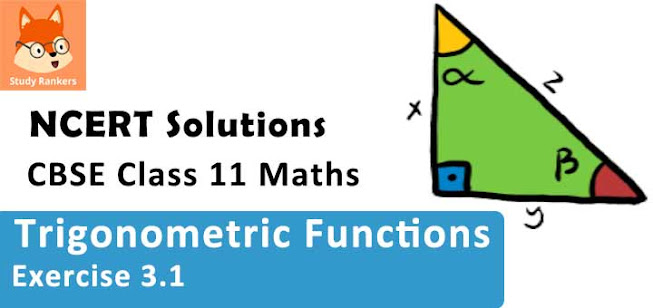### Trigonometric Functions Exercise 3.1 Solutions

1. Find the radian measures corresponding to the following degree measures:
(i) 25°
(ii) –47° 30'
(iii) 240°
(iv) 520°

Solution

(i) 25°
We know that 180° = π radian

(ii) -47° 30'
- 47° 30' = -47.5 degree [1° = 60']
= -95/2 degree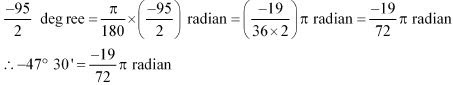(iii) 240°
We know that 180° = π radian

(iv) 520°
We know that 180° = π radian

2. Find the degree measures corresponding to the following radian measures (use π = 22/7).
(i) 11/16
(ii) -4
(iii) 5π/3
(iv) 7π/6

Solution

(i) 11/16
We know that π radian = 180°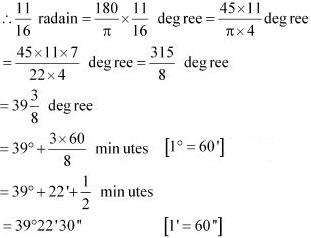(ii) -4
We know that π radian = 180°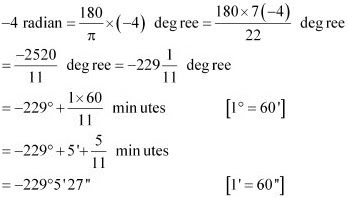(iii) 5π/3
We know that π radian = 180°
∴ 5π/3 radian = 180/π × 5π/3 degree = 300°

(iv) 7π/6
We know that π radian = 180°
∴ 7π/6 radian = 180/π × 7π/6 = 210°

3. A wheel makes 360 revolutions in one minute. Through how many radians does it turn in one second?

Solution

Number of revolutions made by the wheel in 1 minute = 360
∴ Number of revolutions made by the wheel in 1 second = 360/60 = 6
In one complete revolution, the wheel turns an angle of 2π radian.
Hence, in 6 complete revolutions, it will turn an angle of 6 × 2π radian, i.e.,
Thus, in one second, the wheel turns an angle of 12π radian.

4. Find the degree measure of the angle subtended at the centre of a circle of radius 100 cm by an arc of length 22 cm (use π = 22/7) .

Solution

We know that in a circle of radius r unit, if an arc of length l unit subtends an angle θ radian at the centre, then
θ = 1/r
Therefore, for = 100 cm, l = 22 cm, we have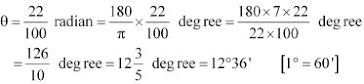Thus, the required angle is 12° 36'.

5. In a circle of diameter 40 cm, the length of a chord is 20 cm. Find the length of minor arc of the chord.

Solution

Diameter of the circle = 40 cm
∴ Radius (r) of the circle = 40/2 cm = 20 cm
Let AB be a chord (length = 20 cm) of the circle.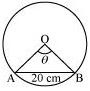In ΔOAB, OA = OB = Radius of circle = 20 cm
Also, AB = 20 cm
Thus, ΔOAB is an equilateral triangle.
∴θ = 60° = π/3 radian
We know that in a circle of radius r unit, if an arc of length l unit subtends an angle θ radian at the centre, then θ = l/r.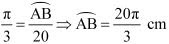Thus, the length of the minor arc of the chord is 20π/3 cm.

6. If in two circles, arcs of the same length subtend angles 60° and 75° at the centre, find the ratio of their radii.

Solution

Let the radii of the two circles be and . Let an arc of length l subtend an angle of 60° at the centre of the circle of radius r1, while let an arc of length subtend an angle of 75° at the centre of the circle of radius r2.
We know that in a circle of radius r unit, if an arc of length l unit subtends an angle θ radian at the centre, then θ = 1/r or l = rθ .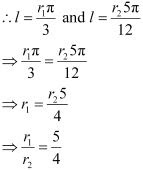Thus, the ratio of the radii is 5 : 4.

7. Find the angle in radian though which a pendulum swings if its length is 75 cm and the tip describes an arc of length
(i) 10 cm
(ii) 15 cm
(iii) 21 cm

Solution

We know that in a circle of radius r unit, if an arc of length l unit subtends an angle θ radian at the centre, then θ = l/r.
It is given that r = 75 cm
(i) Here, l = 10 cm